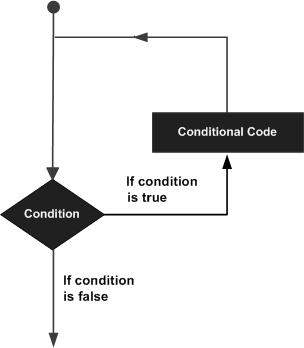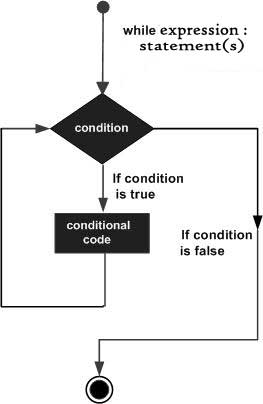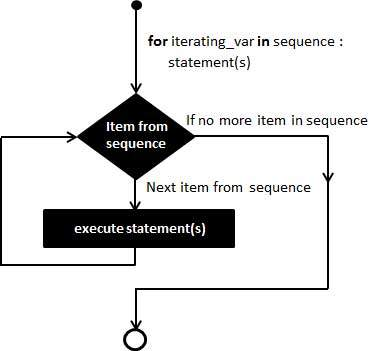# Python 3 Loop Statements: For Loop and While Loop

A loop statement allows us to execute a statement or group of statements multiple times. The following diagram illustrates a loop statement:Python programming language provides the following types of loops to handle looping requirements.

• `while` loops
• `for` loops

## While Loops

A `while` loop statement in Python programming language repeatedly executes a target statement as long as a given condition is true.

### Syntax

The syntax of a while loop in Python programming language is

``````while expression:
statement(s)``````

For example:

``````# Prints out 0,1,2,3,4

count = 0
while count < 5:
print(count)
count += 1  # This is the same as count = count + 1``````

Here, `statement(s)` may be a single statement or a block of statements with uniform indent. The condition may be any expression, and true is any non-zero value. The loop iterates while the condition is true.

When the condition becomes false, program control passes to the line immediately following the loop.

In Python, all the statements indented by the same number of character spaces after a programming construct are considered to be part of a single block of code. Python uses indentation as its method of grouping statements.

### Flow DiagramHere, a key point of the while loop is that the loop might not ever run. When the condition is tested and the result is false, the loop body will be skipped and the first statement after the while loop will be executed.

### `while` loop with `else`

The `else` part is executed if the condition in the while loop evaluates to `False`.

The while loop can be terminated with a `break` statement. In such case, the `else` part is ignored. Hence, a while loop's `else` part runs if no break occurs and the condition is false.

Here is an example to illustrate this.

``````# Example to illustrate
# the use of else statement
# with the while loop

counter = 0

while counter < 3:
print("Inside loop")
counter = counter + 1
else:
print("Inside else")``````

The result will be as follows:

``````Inside loop
Inside loop
Inside loop
Inside else``````

Here, we use a counter variable to print the string `Inside loop` three times.

On the forth iteration, the condition in while becomes `False`. Hence, the `else` part is executed.

### The Infinite Loop

A loop becomes infinite loop if a condition never becomes FALSE. You must be cautious when using while loops because of the possibility that this condition never resolves to a FALSE value. This results in a loop that never ends. Such a loop is called an infinite loop.

An infinite loop might be useful in client/server programming where the server needs to run continuously so that client programs can communicate with it as and when required.

Here is an example:

``````#!/usr/bin/python3

var = 1
while var == 1 :  # This constructs an infinite loop
num = int(input("Enter a number  :"))
print ("You entered: ", num)

print ("Good bye!")``````

When the above code is executed, it produces the following result:

``````Enter a number  :20
You entered:  20
Enter a number  :29
You entered:  29
Enter a number  :3
You entered:  3
Enter a number  :11
You entered:  11
Enter a number  :22
You entered:  22
Enter a number  :Traceback (most recent call last):
File "examples\test.py", line 5, in
num = int(input("Enter a number  :"))
KeyboardInterrupt``````

The above example goes in an infinite loop and you need to use `CTRL+C` to exit the program.

### Single Statement Suites

Similar to the if statement syntax, if your `while` clause consists only of a single statement, it may be placed on the same line as the while header.

Here is the syntax and example of a one-line while clause:

``````#!/usr/bin/python3

flag = 1
while (flag): print ('Given flag is really true!')
print ("Good bye!")``````

## For Loops

For loops iterate over a given sequence.

### Syntax

``````for iterating_var in sequence:
statements(s)``````

Here is an example:

``````primes = [2, 3, 5, 7]
for prime in primes:
print(prime)``````

### Flow Diagram### The range() function

The built-in function `range()` is the right function to iterate over a sequence of numbers. It generates an iterator of arithmetic progressions. For example:

``````>>> range(5)
range(0, 5)
>>> list(range(5))
[0, 1, 2, 3, 4]``````

`range()` generates an iterator to progress integers starting with 0 upto n-1. To obtain a list object of the sequence, it is typecasted to `list()`. Now this list can be iterated using the for statement.

``````>>> for var in list(range(5)):
print (var)``````

This will produce the following output:

``````0
1
2
3
4``````

### Using `else` Statement with Loops

Same as that of `while` loop, we can have an optional `else` block with `for` loop as well. For example:

``````#!/usr/bin/python3

numbers = [11,33,55,39,55,75,37,21,23,41,13]

for num in numbers:
if num%2 == 0:
print ('the list contains an even number')
break
else:
print ('the list doesnot contain even number')``````

When the above code is executed, it produces the following result:

``the list does not contain even number``

## Break Statement

In Python, the `break` statement provides you with the opportunity to exit out of a loop when an external condition is triggered. You'll put the `break` statement within the block of code under your loop statement, usually after a conditional `if` statement.

Let's look at an example that uses the `break` statement in a `for` loop:

``````number = 0

for number in range(10):
number = number + 1

if number == 5:
break    # break here

print('Number is ' + str(number))

print('Out of loop')``````

When we run this code, our output will be the following:

``````Number is 1
Number is 2
Number is 3
Number is 4
Out of loop``````

This shows that once the integer `number` is evaluated as equivalent to 5, the loop breaks, as the program is told to do so with the `break` statement.

The `break` statement causes a program to break out of a loop.

## Continue Statement

The `continue` statement gives you the option to skip over the part of a loop where an external condition is triggered, but to go on to complete the rest of the loop. That is, the current iteration of the loop will be disrupted, but the program will return to the top of the loop.

The `continue` statement will be within the block of code under the loop statement, usually after a conditional `if` statement. For example:

``````number = 0

for number in range(10):
number = number + 1

if number == 5:
continue    # continue here

print('Number is ' + str(number))

print('Out of loop')``````

The difference in using the `continue` statement rather than a `break` statement is that our code will continue despite the disruption when the variable `number` is evaluated as equivalent to 5. Let's look at our output:

``````Number is 1
Number is 2
Number is 3
Number is 4
Number is 6
Number is 7
Number is 8
Number is 9
Number is 10
Out of loop``````

Here we see that the line `Number is 5` never occurs in the output, but the loop continues after that point to print lines for the numbers 6-10 before leaving the loop.

You can use the `continue` statement to avoid deeply nested conditional code, or to optimize a loop by eliminating frequently occurring cases that you would like to reject.

The `continue` statement causes a program to skip certain factors that come up within a loop, but then continue through the rest of the loop.

## Pass Statement

When an external condition is triggered, the `pass` statement allows you to handle the condition without the loop being impacted in any way; all of the code will continue to be read unless a `break` or other statement occurs.

As with the other statements, the `pass` statement will be within the block of code under the loop statement, typically after a conditional `if` statement.

Using the same code block as above, let's replace the `break` or `continue` statement with a `pass` statement:

``````number = 0

for number in range(10):
number = number + 1

if number == 5:
pass    # pass here

print('Number is ' + str(number))

print('Out of loop')``````

The `pass` statement occurring after the `if` conditional statement is telling the program to continue to run the loop and ignore the fact that the variable `number` evaluates as equivalent to 5 during one of its iterations.

We'll run the program and take a look at the output:

``````Number is 1
Number is 2
Number is 3
Number is 4
Number is 5
Number is 6
Number is 7
Number is 8
Number is 9
Number is 10
Out of loop``````

By using the `pass` statement in this program, we notice that the program runs exactly as it would if there were no conditional statement in the program. The `pass` statement tells the program to disregard that condition and continue to run the program as usual.

The `pass` statement can create minimal classes, or act as a placeholder when working on new code and thinking on an algorithmic level before hammering out details.

## Iterator and Generator

`Iterator` is an object which allows a programmer to traverse through all the elements of a collection, regardless of its specific implementation. In Python, an iterator object implements two methods, `iter()` and `next()`.

String, List or Tuple objects can be used to create an Iterator.

``````list = [1,2,3,4]
it = iter(list) # this builds an iterator object
print (next(it)) #prints next available element in iterator
Iterator object can be traversed using regular for statement
!usr/bin/python3
for x in it:
print (x, end=" ")
or using next() function
while True:
try:
print (next(it))
except StopIteration:
sys.exit() #you have to import sys module for this``````

A generator is a function that produces or yields a sequence of values using yield method.

When a generator function is called, it returns a generator object without even beginning execution of the function. When the `next()` method is called for the first time, the function starts executing until it reaches the yield statement, which returns the yielded value. The yield keeps track i.e. remembers the last execution and the second `next()` call continues from previous value.

The following example defines a generator, which generates an iterator for all the Fibonacci numbers.

``````#!usr/bin/python3

import sys
def fibonacci(n): #generator function
a, b, counter = 0, 1, 0
while True:
if (counter > n):
return
yield a
a, b = b, a + b
counter += 1
f = fibonacci(5) #f is iterator object

while True:
try:
print (next(f), end=" ")
except StopIteration:
sys.exit()``````

## Conclusion

In this tutorial, we learned a lot about python loops and learned to use break, continue, pass statements in loop statements. Next, we will learn some basic data types deeply in the further sections.

## 1 Comment

temp

#typo in section “Using else Statement with Loops”, missing space in 1st sample code:

else:   print ('the list doesnot contain even number')

#should be

else:   print ('the list does not contain even number')